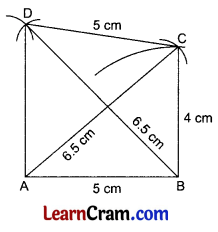# DAV Class 8 Maths Chapter 12 Worksheet 2 Solutions

The DAV Maths Class 8 Solutions and DAV Class 8 Maths Chapter 12 Worksheet 2 Solutions of Construction of Quadrilaterals offer comprehensive answers to textbook questions.

## DAV Class 8 Maths Ch 12 WS 2 Solutions

Question 1.
Construct a quadrilateral ABCD in which $$\overline{\mathrm{AB}}$$ = 4 cm, $$\overline{\mathrm{BC}}$$ = 3 cm, $$\overline{\mathrm{CD}}$$ = 3.5 cm and diagonals are $$\overline{\mathrm{AC}}$$ = 6 cm and $$\overline{\mathrm{BD}}$$ = 5.5 cm.
Solution:
Steps of Construction:
1. Draw $$\overline{\mathrm{AB}}$$ = 4 cm.
2. Draw arcs from A and B with radii 6 cm and 3 cm respectively to meet at C.
3. Join $$\overline{\mathrm{AC}}$$ and $$\overline{\mathrm{BC}}$$.
4. Draw arcs from C and B with radii 3.5 cm and 5.5 cm respectively to meet at D.
5. Join $$\overline{\mathrm{CD}}$$, $$\overline{\mathrm{BD}}$$ and $$\overline{\mathrm{AD}}$$.
6. ABCD is the required quadrilateral.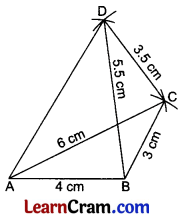Question 2.
Construct a quadrilateral ABCD in which $$\overline{\mathrm{AB}}$$ = $$\overline{\mathrm{BC}}$$ = 5 cm, $$\overline{\mathrm{CD}}$$ = 6 cm, diagonals are 7 cm each.
Solution:
Steps of Construction:
1. Draw $$\overline{\mathrm{AB}}$$ = 5 cm.
2. Draw arcs from A and B with radius 7 cm and 5 cm respectively.
3. Join $$\overline{\mathrm{AC}}$$ and $$\overline{\mathrm{BC}}$$.
4. Draw arcs from C and B with centres 6 cm and 7 cm respectively.
5. Join $$\overline{\mathrm{BD}}$$ and $$\overline{\mathrm{CD}}$$.
6. ABCD is the required quadrilateral.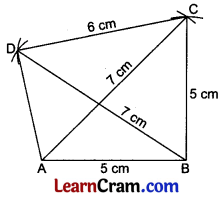Question 3.
Construct a quadrilateral ABCD in which three sides are 4 cm each and both diagonals are 6 cm each.
Solution:
Steps of Construction:
1. Draw $$\overline{\mathrm{AB}}$$ = 4 cm.
2. Draw arcs from A and B with radii 6 cm and 4 cm respectively to meet at C.
3. Join $$\overline{\mathrm{AC}}$$ and $$\overline{\mathrm{BC}}$$.
4. Draw arcs from C and B with radii 4 cm and 6 cm respectively to meet at D.
5. Join $$\overline{\mathrm{BD}}$$ and $$\overline{\mathrm{CD}}$$.
6. Join $$\overline{\mathrm{AD}}$$.
7. ABCD is the required quadrilateral.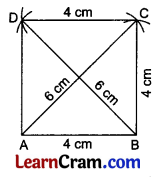Question 4.
Construct a quadrilateral MNRS with sides $$\overline{\mathrm{MN}}$$ = 5.5 cm, $$\overline{\mathrm{NR}}$$ = 6 cm, $$\overline{\mathrm{RS}}$$ = 6.5 cm and diagonals $$\overline{\mathrm{MR}}$$ = 8 cm and $$\overline{\mathrm{NS}}$$ = 7.5 cm.
Solution:
Steps of Construction:
1. Draw $$\overline{\mathrm{MN}}$$ = 5.5 cm.
2. Draw arcs from M and N with radii 8 cm and 6 cm respectively to meet at R.
3. Join $$\overline{\mathrm{MR}}$$ and $$\overline{\mathrm{NR}}$$.
4. Draw arcs from N and R with radii 7.5 cm and 6.5 cm respectively to meet at S.
5. Join $$\overline{\mathrm{NS}}$$, $$\overline{\mathrm{RS}}$$ and $$\overline{\mathrm{MS}}$$.
6. MNRS is the required quadrilateral.Question 5.
Construct a quadrilateral ABCD in which $$\overline{\mathrm{AB}}$$ = 4.5 cm, $$\overline{\mathrm{BC}}$$ = 4 cm, $$\overline{\mathrm{CD}}$$ = 4.5 cm, diagonals $$\overline{\mathrm{AC}}$$ = 6 cm and $$\overline{\mathrm{BD}}$$ = 6.5 cm.
Solution:
Steps of Construction:
1. Draw $$\overline{\mathrm{AB}}$$ = 4.5 cm.
2. Draw arcs from A and B with radii 6 cm and 4 respectively to meet at C.
3. Join $$\overline{\mathrm{AC}}$$ and $$\overline{\mathrm{BC}}$$.
4. Draw arcs from B and C with radii 6.5 cm and 4.5 c respectively to meet at D.
5. Join $$\overline{\mathrm{CD}}$$, $$\overline{\mathrm{BD}}$$ and $$\overline{\mathrm{AD}}$$.
6. ABCD is the required quadrilateral.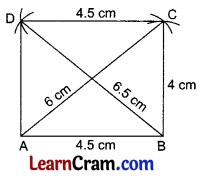Question 6.
Construct a quadrilateral ABCD in which $$\overline{\mathrm{AB}}$$ = 5 cm, $$\overline{\mathrm{BC}}$$ = 4 cm, $$\overline{\mathrm{CD}}$$ = 5 cm, diagonals $$\overline{\mathrm{BD}}$$ = $$\overline{\mathrm{AC}}$$ = 6.5 cm.
Solution:
Steps of Construction:
1. Draw a line segment $$\overline{\mathrm{AB}}$$ = 5 cm.
2. Draw arcs from A and B by taking a 6.5 cm radius for each.
3. Draw an arc from B by taking a 4 cm radius intersecting the previous arc at C.
4. Take a radius of 5 cm and draw an arc from C to intersect the previous arc at D.
5. Join $$\overline{\mathrm{AC}}$$, $$\overline{\mathrm{BD}}$$, $$\overline{\mathrm{CD}}$$ and $$\overline{\mathrm{AD}}$$.
6. ABCD is the required quadrilateral.